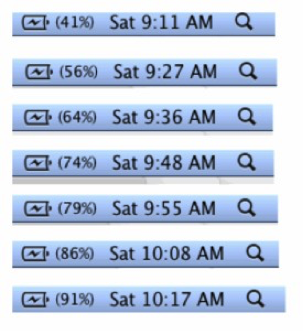# Descriptive Statistics

## Objective

Describe the relationship between two quantitative variables in a contextual situation represented in a scatterplot using the correlation coefficient, least squares regression, and residuals as evidence.

## Common Core Standards

### Core Standards

?

• HSS-ID.B.6a — Fit a function to the data; use functions fitted to data to solve problems in the context of the data. Use given functions or choose a function suggested by the context. Emphasize linear, quadratic, and exponential models.

• HSS-ID.C.7 — Interpret the slope (rate of change) and the intercept (constant term) of a linear model in the context of the data.

• HSS-ID.C.9 — Distinguish between correlation and causation.

## Criteria for Success

?

1. Describe bivariate by the shape (function of best fit, as determined by the residuals), center (equation of line or function of best fit), and spread (correlation coefficient and analysis of residuals).
2. Analyze bivariate data to describe a plausible relationship using information about a set of data's linearity, correlation, line of best fit, and related residuals.
3. Explain that correlation does not indicate causality, so one variable cannot be determined to cause another.
4. Understand that in a scatterplot, there is the actual data (points) and the prediction (equation modeling the line or function of best fit).
5. Make predictions about the contextual situation based on the least squares regression line.

## Tips for Teachers

?

• This lesson provides students with focused practice on all of the skills they will need to complete the project in lessons 20–22.
• There are only three problems referenced in this lesson frame because of the length of each problem. Due to the fact that the problem set guidance problems are performance tasks, there is no target task.
• This lesson provides an opportunity for bivariate review of concepts learned in this section of the unit before students embark on a project.

## Anchor Problems

?

Jerry forgot to plug in his laptop before he went to bed. He wants to take the laptop to his friend’s house with a full battery. The pictures below show the screenshots of the battery charge indicator after he plugs in the computer.• Represent this information on a scatterplot.
• Determine the correlation coefficient for this data to determine the strength of association.
• Assign a line of best fit either through least squares regression or estimation.
• Determine if a linear function is the best model for this data through plotting the residuals.

Using your analysis of this data, make an estimate: When can Jerry expect that his laptop is fully charged?

#### References

Illustrative Mathematics Laptop Battery Charge 2

Laptop Battery Charge 2, accessed on June 21, 2017, 11:44 a.m., is licensed by Illustrative Mathematics under either the CC BY 4.0 or CC BY-NC-SA 4.0. For further information, contact Illustrative Mathematics.

## Problem Set

?

The following resources include problems and activities aligned to the objective of the lesson that can be used to create your own problem set.

• The following two problems from Illustrative Mathematics should be treated as performance tasks and should be adapted to be modeled after Anchor Problem #1. Students will need to incorporate all of the skills used to analyze bivariate data when they work on a project in the next three lessons.